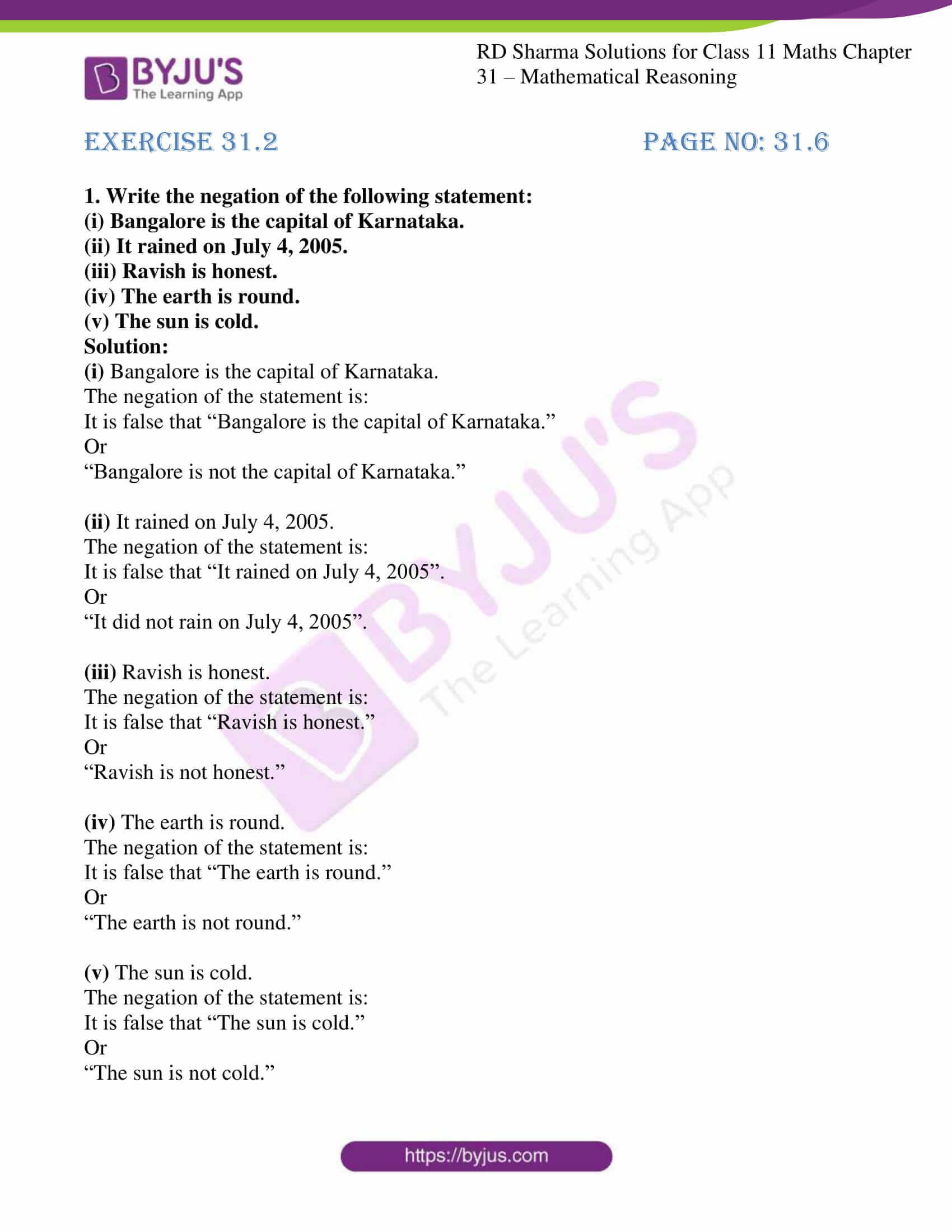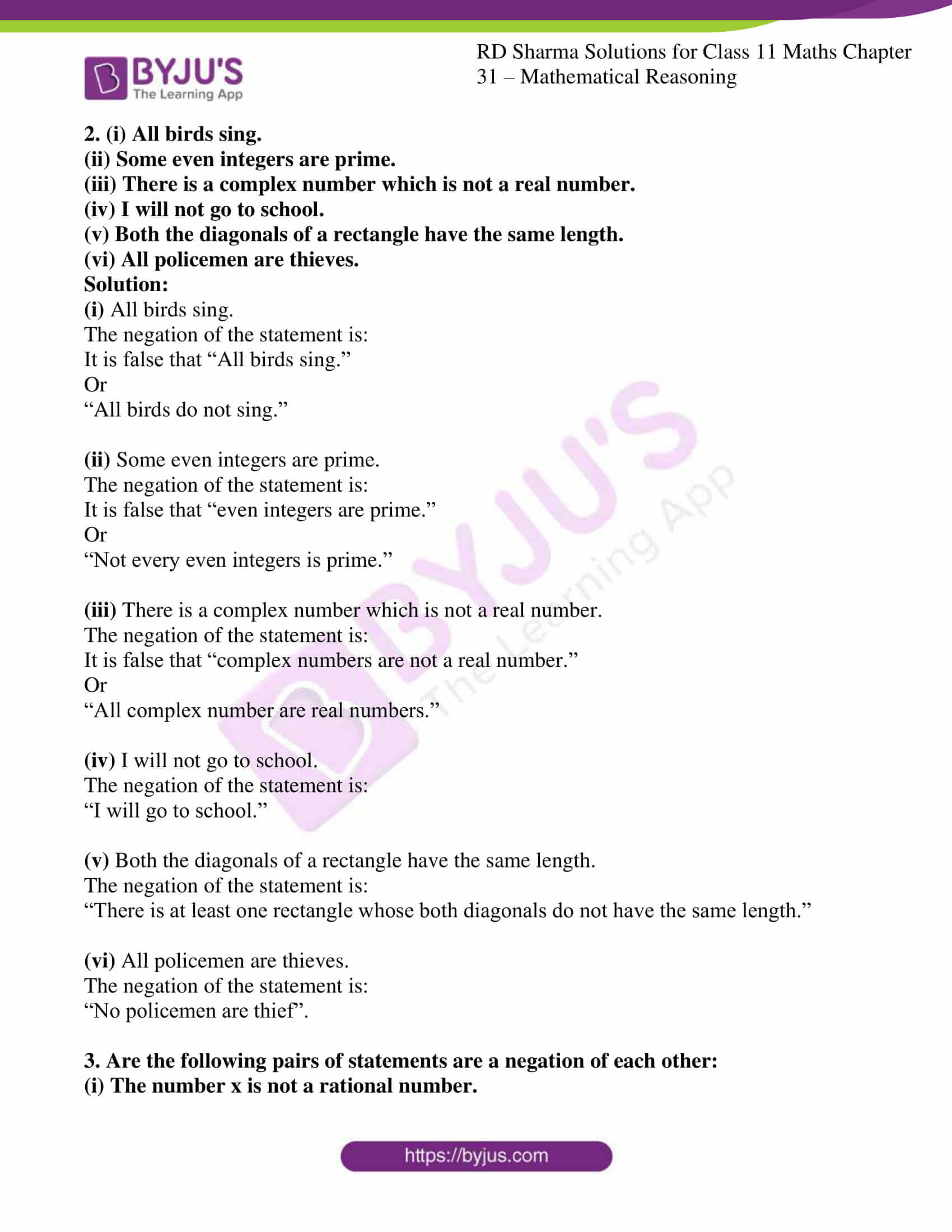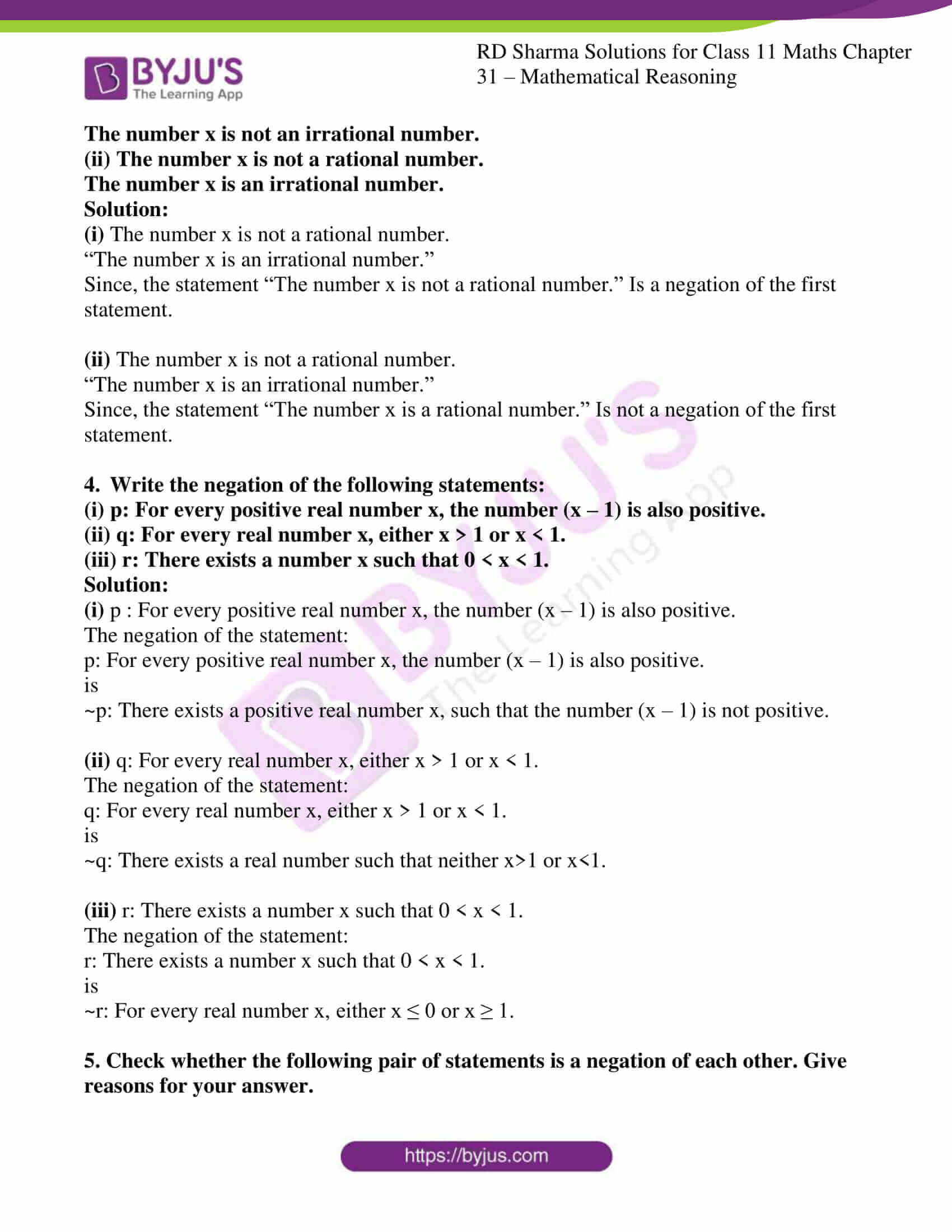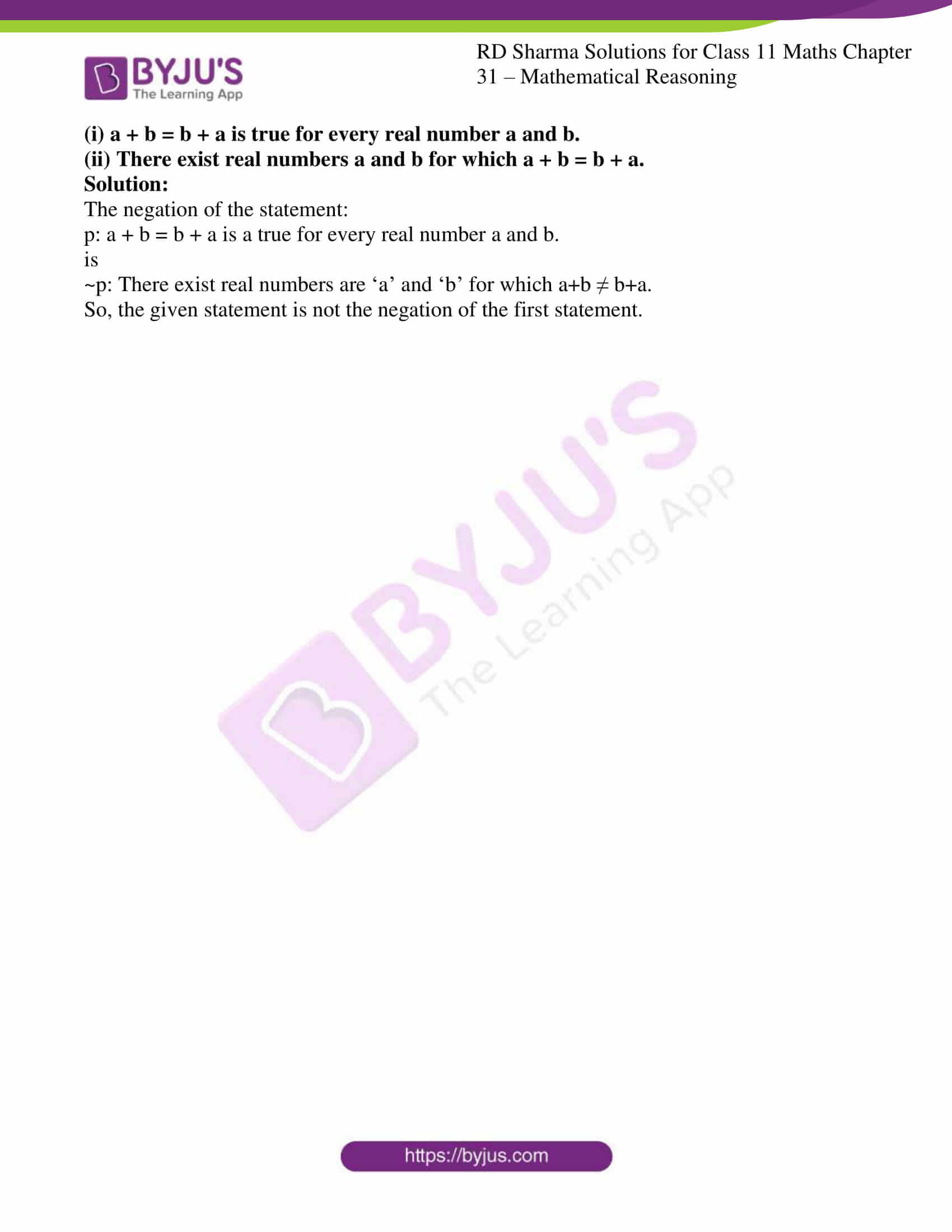# RD Sharma Solutions for Class 11 Chapter 31 - Mathematical Reasoning Exercise 31.2

“The denial of a statement is termed as negation of a statement”. In this exercise, we shall discuss the concept negation of a statement. The solutions are developed by teachers at BYJU’S having vast experience in the education industry. The main aim of providing solutions is to help students, irrespective of their intelligence quotient. The solutions here are explained in simple language to help students understand the concepts easily and perform well in the annual exam. Students are advised to solve the exercise wise problems on a daily basis to understand the concepts in a better way. Students can download RD Sharma Class 11 Maths Solutions from the links given below and can start practising offline.

## Download the pdf of RD Sharma Solutions for Class 11 Maths Exercise 31.2 Chapter 31 – Mathematical Reasoning### Access answers to RD Sharma Solutions for Class 11 Maths Exercise 31.2 Chapter 31 – Mathematical Reasoning

#### EXERCISE 31.2 PAGE NO: 31.6

1. Write the negation of the following statement:
(i) Bangalore is the capital of Karnataka.

(ii) It rained on July 4, 2005.

(iii) Ravish is honest.

(iv) The earth is round.

(v) The sun is cold.

Solution:

(i) Bangalore is the capital of Karnataka.

The negation of the statement is:

It is false that “Bangalore is the capital of Karnataka.”

Or

“Bangalore is not the capital of Karnataka.”

(ii) It rained on July 4, 2005.

The negation of the statement is:

It is false that “It rained on July 4, 2005”.

Or

“It did not rain on July 4, 2005”.

(iii) Ravish is honest.

The negation of the statement is:

It is false that “Ravish is honest.”

Or

“Ravish is not honest.”

(iv) The earth is round.

The negation of the statement is:

It is false that “The earth is round.”

Or

“The earth is not round.”

(v) The sun is cold.

The negation of the statement is:

It is false that “The sun is cold.”

Or

“The sun is not cold.”

2. (i) All birds sing.

(ii) Some even integers are prime.

(iii) There is a complex number which is not a real number.

(iv) I will not go to school.

(v) Both the diagonals of a rectangle have the same length.

(vi) All policemen are thieves.

Solution:

(i) All birds sing.

The negation of the statement is:

It is false that “All birds sing.”

Or

“All birds do not sing.”

(ii) Some even integers are prime.

The negation of the statement is:

It is false that “even integers are prime.”

Or

“Not every even integers is prime.”

(iii) There is a complex number which is not a real number.

The negation of the statement is:

It is false that “complex numbers are not a real number.”

Or

“All complex number are real numbers.”

(iv) I will not go to school.

The negation of the statement is:

“I will go to school.”

(v) Both the diagonals of a rectangle have the same length.

The negation of the statement is:

“There is at least one rectangle whose both diagonals do not have the same length.”

(vi) All policemen are thieves.

The negation of the statement is:

“No policemen are thief”.

3. Are the following pairs of statements are a negation of each other:
(i) The number x is not a rational number.
The number x is not an irrational number.
(ii) The number x is not a rational number.
The number x is an irrational number.

Solution:

(i) The number x is not a rational number.

“The number x is an irrational number.”

Since, the statement “The number x is not a rational number.” Is a negation of the first statement.

(ii) The number x is not a rational number.

“The number x is an irrational number.”

Since, the statement “The number x is a rational number.” Is not a negation of the first statement.

4. Write the negation of the following statements:

(i) p: For every positive real number x, the number (x – 1) is also positive.

(ii) q: For every real number x, either x > 1 or x < 1.

(iii) r: There exists a number x such that 0 < x < 1.

Solution:

(i) p : For every positive real number x, the number (x – 1) is also positive.

The negation of the statement:

p: For every positive real number x, the number (x – 1) is also positive.

is

~p: There exists a positive real number x, such that the number (x – 1) is not positive.

(ii) q: For every real number x, either x > 1 or x < 1.

The negation of the statement:

q: For every real number x, either x > 1 or x < 1.

is

~q: There exists a real number such that neither x>1 or x<1.

(iii) r: There exists a number x such that 0 < x < 1.

The negation of the statement:

r: There exists a number x such that 0 < x < 1.

is

~r: For every real number x, either x ≤ 0 or x ≥ 1.

5. Check whether the following pair of statements is a negation of each other. Give reasons for your answer.

(i) a + b = b + a is true for every real number a and b.
(ii) There exist real numbers a and b for which a + b = b + a.

Solution:

The negation of the statement:

p: a + b = b + a is a true for every real number a and b.

is

~p: There exist real numbers are ‘a’ and ‘b’ for which a+b ≠ b+a.

So, the given statement is not the negation of the first statement.